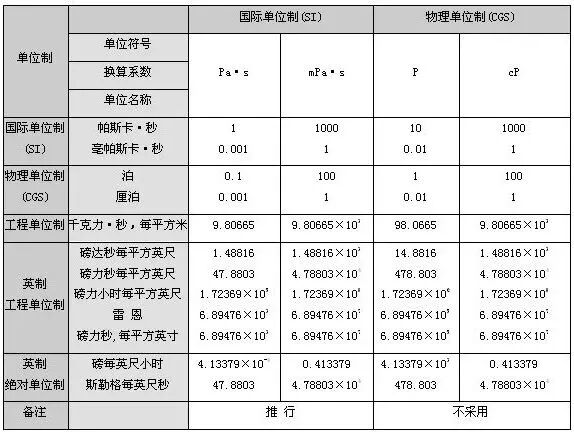## 服务与支持

### 服务与支持

【知识分享】实验室常用单位换算公式大全，果断收藏！1 ppm=1000 ppb

1 ppb=1000 ppt
ppm即：mg/L（毫克/升）
ppb即：μg/L（微克/升）
ppt即：ng/L（纳克/升）

（一）溶液的浓度

01

02

（1）摩尔浓度

（2）当量浓度(N)

03

04

1）当量浓度=1000.d.质量百分浓度/E
2）质量百分浓度=当量浓度E/1000.d
3）摩尔浓度=1000.d质量百分浓度/M
4）质量百分浓度=质量-体积浓度（毫克/升）/104.d 5）质量-体积浓度（mg/L）=104质量百分浓度

05

ppm是重量的百分率

ppm=mg/kg=mg/L

1 ppb=1 μg/L=0.001 mg

d—溶液的比重；

M—溶质的摩尔质量；

（二）气体浓度

01

1 ppm=10-6=一百万分之一,1 ppb=10-9=十亿分之一

1 ppt=10-12=万亿分之一,1 ppm=10³ppb=10²10²10²ppt

02

C=22.4X/M

C—污染物以ppm表示的浓度值
M—污染物的分子量

1 ppm=M/22.4(mg/m3)=1000×M/22.4(μg/m3)

03

1 ppm=1 mg/kg=1000 μg/kg

1 ppb=1 μg/kg=10-3 mg/kg

1 mg/kg=1 ppm=1000 μg/kg

1 μg/kg=1 ppb=10-3 ppm

1千米(km)=0.621英里(mile)

1米(m)=3.281英尺(ft)=1.094码(yd)

1厘米(cm)=0.394英寸(in)

1英里(mile)=1.609千米(km)

1英尺(ft)=0.3048米(m)

1英寸(in)=2.54厘米(cm)

1海里(n mile)=1.852千米(km)

1码(yd)=0.9144米(m)

1英尺(ft)=12英寸(in)

1码(yd)=3英尺(ft)

1英里(mile)=5280英尺(ft)

1海里(n mile)=1.1516英里(mile)

1吨(t)=1000千克(kg)=2205磅(lb)=1.102短吨(sh.ton)=0.934长吨(long.ton)

1千克(kg)=2.205磅(lb)1短吨(sh.ton)=0.907吨(t)=2000磅(1b)

1长吨(long.ton)=1.016吨(t)

1磅(lb)=0.454千克(kg)

1盎司(oz)=28.350克(g)

1千克/米3(kg/m3)=0.001克/厘米3(g/cm3)=0.0624磅/英尺3(lb/ft3)

1磅/英尺3(lb/ft3)=16.02千克/米3(kg/m3)

1磅/英寸3(lb/in3)=27679.9千克/米3(kg/m3)

1磅/美加仑(lb/gal)=119.826千克/米3(kg/m3)

1磅/英加仑(lb/gal)=99.776千克/米3(kg/m3)

1磅/(石油)桶(lb/bbl)=2.853千克/米3(kg/m3)

1波美密度=140/15.5℃时的比重-130API=141.5/15.5℃时的比重-131.5

1兆帕(MPa)=145磅/英寸2(psi)=10.2千克/厘米2(kg/cm2)=10巴(bar)=9.8大气压(at m)

1磅/英寸2(psi)=0.006895兆帕(MPa)=0.0703千克/厘米2(kg/cm2)=0.0689巴(bar)=0.068大气压(at m)
1巴(bar)=0.1兆帕(MPa)=14.503磅/英寸2(psi)=1.0197千克/厘米2(kg/cm2)=0.987大气压(at m)
1大气压(at m)=0.101325兆帕(MPa)=14.696磅/英寸2(psi)=1.0333千克/厘米2(kg/cm2)
=1.0133巴(bar)

1平方公里(km2)=100公顷(ha)=247.1英亩(acre)=0.386平方英里(mile2)

1平方米(m2)=10.764平方英尺(ft2)

1公亩(acre)=100平方米(m2)

1公顷(ha)=10000平方米(m2)=2.471英亩(acre)

1平方英里(mile2)=2.590平方公里(km2)

1英亩(acre)=0.4047公顷(ha)=0.0040469平方公里(km2)=4047平方米(m2)

1平方英尺(ft2)=0.093平方米(m2)

1平方英寸(in2)=6.452平方厘米(cm2)

1平方码(yd2)=0.8361平方米(m2)

1立方米(m3)=1000升(liter)=35.315立方英尺(ft3)=6.290桶(bbl)

1立方英尺(ft3)=0.0283立方米(m3)=28.317升(l)

1千立方英尺(mcf)=28.317立方米(m3)

1百万立方英尺(MMcf)=2.8317万立方米(m3)

10亿立方英尺(bcf)=2831.7万立方米(m3)

1万亿立方英尺(tcf)=283.17亿立方米(m3)

1立方英寸(in3)=16.3871立方厘米(cm3)

1英亩·英尺=1234立方米(m3)

1桶(bbl)=0.159立方米(m3)=42美加仑(gal)

1美加仑(gal)=3.785升(l)

1美夸脱(qt)=0.946升(l)

1美品脱(pt)=0.473升(l)

1美吉耳(gi)=0.118升(l)

1英加仑(gal)=4.546升(l)

1英尺2/秒(ft2/s)=9.29030*10-22/秒(m2/s)

1斯(St)=10-42/秒(m2/s)

1厘斯(eSt)=10-62/秒(m2/s)=1毫米2/秒(mm2/s)

1泊 (1P)=100厘泊(100cP)

1厘泊(1cP)=1毫帕斯卡·秒 (1mPa·s)
1毫帕斯卡·秒 (1 mPa·s)=1000微 帕斯卡·秒（1000 μ Pa.s）1牛顿(N)=0.225磅力(1bf)=0.102千克力(kgf)=10^5达因(dyn)

1千克力(kgf)=9.81牛顿(N)

1磅力(1bf)=4.45牛顿(N)

1达因(dyn)=1克*厘米/秒^2

K(开尔文度)=5/9(℉+459.67)K=℃+273.15n℉=[(n-32)*5/9]℃n℃(摄氏度)=(5/9·n+32)℉1℉(华氏度)=5/9℃(温度差)

1千卡(米2·时)(kcal/m2·h)=1.16279瓦/米2(W/m2)

1千卡/（米2 ·时·℃）〔1kcal/(m2 ·h·℃)〕=1.16279瓦/（米2 ·开尔文）〔w/(m2 ·K)〕

1英热单位/（英尺2 ·时·°F）〔Btu/(ft2 ·h·°F)〕=5.67826瓦/（米2 ·开尔文）〔（w/m2 ·K）〕

1米2 ·时·℃/千卡（m2 ·h·℃/kcal）=0.86000米2 ·开尔文/瓦（m2 ·K/W）

1千卡/(千克·℃)[kcal/(kg·℃)]=1英热单位/(磅·℉)[Btu/(lb·℉)]=4186.8焦耳/(千克·开尔文)[J/(kg·K)]

1焦耳=0.10204千克·米=2.778*10-7千瓦·小时=3.777*10-7公制马力小时=3.723*10-7英制马力小时=2.389*10-4千卡=9.48*10-4英热单位

1卡(cal)=4.1868焦耳(J)

1英热单位(Btu)=1055.06焦耳(J)

1千克力米(kgf·m)=9.80665焦耳(J)

1英尺磅力(ft·1bt)=1.35582焦耳(J)

1米制马力小时(hp·h)=2.64779*106焦耳(J)

1英制马力小时(UKHp·h)=2.68452*106焦耳(J)

1千瓦小时(kw·h)=3.6*106焦耳(J)

1大卡=4186.75焦耳(J)

1千克力·米/秒(kgf·m/s)=9.80665瓦(W)

1米制马力(hp)=735.499瓦(W)

1卡/秒(cal/s)=4.1868瓦(W)

1英热单位/时(Btu/h)=0.293071瓦(W)

1英尺/秒(ft/s)=0.3048米/秒(m/s)

1英里/时(mile/h)=0.44704米/秒(m/s）